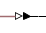Convert physical signal into Simulink output signal

•Libraries:
Simscape / Utilities

## Description

The PS-Simulink Converter block converts a physical signal into a Simulink® output signal. Use this block to connect outputs of a Simscape™ physical network to Simulink scopes or other Simulink blocks.

### Block Icon Display on the Model Canvas

To convey signal conversion while taking up minimal canvas space, the block icon changes dynamically based on whether it is connected to other blocks.

When Block Is...Block Icon

UnconnectedConnected to other blocks### Unit Conversion and Checking

The Output signal unit parameter lets you specify the desired units for the output signal. These units must be commensurate with the units of the input physical signal coming into the block. If you specify a desired output unit, the block applies a gain equal to the conversion factor before outputting the Simulink signal. For example, if the input physical signal coming into the block is displacement, in meters, and you set Output signal unit to `mm`, the block multiplies the value of the input signal by 1e3 before outputting it. If the output signal unit is the same as the input signal unit, no gain is applied.

The default value of the Output signal unit parameter, `inherit`, automatically sets the unit at the block output port to match the unit of the input physical signal coming into the block, based on unit propagation rules. This way, you can easily connect a PS-Simulink Converter block to any signal, without worrying about setting the commensurate output unit.

In the diagram below, the input signal for the PS-Simulink Converter block is torque in N*m, and if you do not specify the output signal unit, the Display block shows the value of 10. If you change the Output signal unit parameter value in the PS-Simulink Converter block to `N*cm`, the torque value in the Display block changes to 1000, as shown in the diagram.When the output signal is related to thermodynamic variables and contains units of temperature, you must decide whether affine conversion needs to be applied. For more information, see When to Apply Affine Conversion. Usually, if the output signal represents a relative temperature, that is, a change in temperature, you need to apply linear conversion, ΔTnew = L * ΔTold (the default method). However, if the output signal represents an absolute temperature, you need to apply affine conversion, Tnew = L * Told + O.

In the following diagram, the Display block shows the room temperature. If you want to display it in degrees Celsius, open the PS-Simulink Converter block, type `degC` in the Output signal unit field, and select the Apply affine conversion check box. The display reading is 24.35. However, if you leave the Apply affine conversion check box clear, the Display block would show 297.5.Note

Unit specified for the output signal by using the Output signal unit parameter does not propagate outside of the physical network. However, if you also specify a physical unit as an attribute of the Simulink signal connected to the output port of the block, the software checks that the two units match. For more information, see Working with Simulink Units.

## Ports

### Input

expand all

Input physical signal that the block converts into the output Simulink signal.

### Output

expand all

Simulink signal that the block outputs after conversion of the input physical signal. The signal size matches the size of the input physical signal. The Output signal unit parameter and Apply affine conversion check box let you apply scaling and linear offset to the input signal value to calculate the correct value of the output signal.

Data Types: `double`

## Parameters

expand all

Specify how to output vector physical signals:

• `inherit` — Format the Simulink output signal to match the format of the physical signal: scalar, row or column vector, or 2-D matrix.

• `1-D array` — If the physical signal is a row or column vector, format the output signal as a Simulink 1-D array. Use this option to simplify plotting of such signals.

Specify the desired units for the output signal. These units must be commensurate with the units of the input physical signal coming into the block. The system compares the units you specified with the actual units of the input physical signal and applies a gain equal to the conversion factor before outputting the Simulink signal. You can select a unit from the drop-down list, or type the desired unit name, such as `rpm`, or a valid expression, such as `mm/s`. For more information and a list of unit abbreviations, see How to Specify Units in Block Dialogs and Unit Definitions. The default value is `inherit`, which means that the output unit matches the unit at the block input port and no gain is applied.

This check box is applicable only for units that can be converted either with or without an affine offset, such as `degC` or `degF`. Select this check box if the output signal represents absolute temperature in degrees Celsius or degrees Fahrenheit. For more information, see Thermal Unit Conversions.

## Version History

Introduced in R2007a

expand all